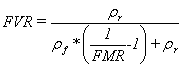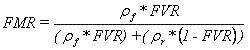# Volume fraction from mass fraction calculation

If you have reached this page on the web and want a volume/mass fraction conversion there is an online calculation tool on this web page.

On the Cadfil utilities menu there is an option to make some calculations using equations useful in filament winding & composites. The user enters the data in the boxes for the calculation required and then clicks the Calculate button to display the answer in the results box. One of the calculation is to calculate Fibre Fraction By Mass from the fibre fraction by volume and Fibre Fraction By Volume from the fibre fraction by Mass.## Mass fraction from volume fraction calculationResin Density ρr

Fibre Density ρf

Mass fraction of fibres in composite. FMR

Volume fraction of fibres in composite. FVR

The units of Resin Density and Fibre Density must be consistent.

Updated: June 2020